The Virial Theorem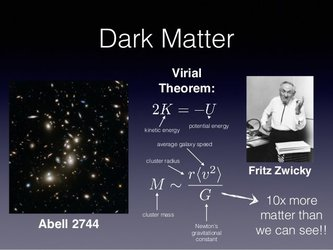In the Gravitation topic, we calculated the energy of a satellite orbiting a planet in a circular orbit as;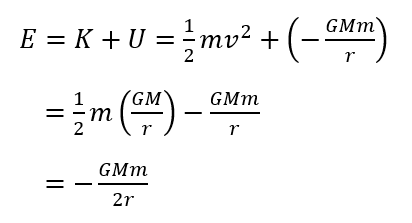where r is the distance between the centers of the planet and the satellite.

It is seen that the total energy is half of the Gravitational potential energy. As we know,This is in fact the statement of the Virial Theorem. But remember we considered the orbit of the satellite to be in circular orbit which isn't generally true. The orbit of the satellite is elliptical in nature. Nevertheless, this theorem is the most fundamental theorem of the universe like Newton's law of motion. Virial Theorem can be stated as, 'For gravitationally bound systems in equilibrium, it can be shown that the total energy is always one-half of the time-averaged potential energy'. Fritz Zwicky, a Swiss astronomer, used this theorem to deduce the existence of the most mysterious matter till now, called dark matter.

In the process of proving the theorem, we consider a quantity as;where vector pi and ri are the linear momentum and position vectors for particle i in some inertial reference frame, and the sum is taken to be overall particles in the system. When we take the time derivative of Q, the equation becomes,We know, Momentum is the product of mass of the particle and its velocityFrom Rotational dynamics, we know the Moment of Inertia is a quantity that expresses the particle's tendency to resist the angular motion. Mathematically, the Moment of Inertia of the collection of particles can be written as,The left-hand side term dQ/dt can also be written as,Putting the above result into equation (i),The second term can be manipulated as below,We get the term is twice the negative the total kinetic energy of the system. From Newton's second law of motion, we know that the rate of change of momentum equals the force exerted by the body.Now in equation (ii), we get,The right-hand side term of the above equation is known as the virial of Clausius, named after the physicist who first found this important energy relation.

Let, Fij is the force of interaction between two particles in the system which on particle i due to particle j, then considering all the forces acting on i,we can rewrite the position vector of particle i as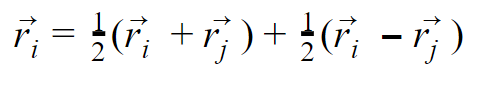We find the above equation as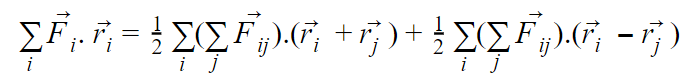From Newton's third law, Fij=-Fji which implies that the first term on the right-hand side is zero by symmetry. The Virial of Clausius may be expressed asWe assume that the only contribution is only from the gravitational interaction between the massive particles included in the system, then Fij  iswhere rij =|rj ﻿-ri| is the separation between i and j, and rij is the unit vector directed from i to j :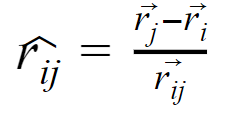Substituting Fij and unit vector rij  in equation (iv),we also know the potential energy between particles i and j,Our  equation  simply becomes,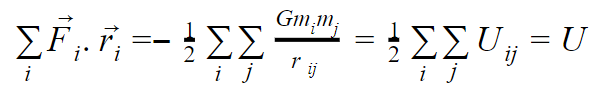Now, substituting the above result to equation (iii) and taking the average with respect to time givesThe average d2I/dt2 over some interval τ can be obtained using the  Mean value theorem of calculus,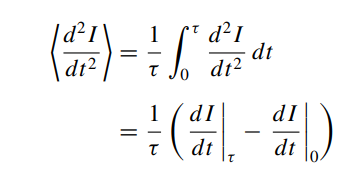If the system is periodic which is the case for orbital motion, thenThen the average over a period will be zero as the system has reached an equilibrium and steady-state configuration which due to which the change in moment of Inertia remains constant.  So the equation becomes,we know total energy is simply the sum of Kinetic energy and Potential energy,then Total energy can be written aswhich proves the Virial theorem.

In short, the Virial theorem relates the time-average of total kinetic energy of a system to that of its potential energy.

It can be used to make a crude estimate of the mass of a cluster of galaxies. Try it yourself for a cluster of spatial extent R and the mean square velocities of the individual galaxies about <V2>.

problem related to this theorem on the hardest test.